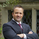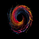3923 görüntülenme
3923
As we know, %b is a measure of where prices are in relation to the outer Bollinger bands and therefore strongly related to volatility . This can get choppy, though.

Vervoort smoothed this using Zero lag TEMA of custom Heiken-ashi recalculated prices. According to Vervoort, "Smoothed Vervoort %b is often a leading indicator making smooth moves with clear turning points. Normal and hidden divergent moves make it an ideal tool to help find entry and exit points while watching price moving between the Bollinger bands ."

List of my other indicators:
- Chart:
```//
// @author LazyBear
// List of all my indicators:
//
study("Vervoort Modified BB%b [LazyBear]", shorttitle="VMBB%b_LB")
calc_tema(s, length) =>
ema1 = ema(s, length)
ema2 = ema(ema1, length)
ema3 = ema(ema2, length)
3 * (ema1 - ema2) + ema3

length=input(18, minval=2, maxval=100, title="%B Length")
temaLength=input(8, title="TEMA Length")
stdevHigh=input(1.6, title="Stdev High")
stdevLow=input(1.6, title="Stdev Low")
stdevLength=input(200, title="Stdev Length")
haOpen=(ohlc4+nz(haOpen))/2
haC=(ohlc4+haOpen+max(high, haOpen)+min(low, haOpen))/4

tma1 = calc_tema(haC,temaLength)
tma2 = calc_tema(tma1, temaLength)
diff = tma1-tma2
zlha = tma1+diff
percb = (calc_tema(zlha,temaLength)+2*stdev(calc_tema(zlha,temaLength),length) - wma(calc_tema(zlha,temaLength),length))/(4*stdev(calc_tema(zlha,temaLength),length))*100

ub=50+stdevHigh*stdev(percb,stdevLength)
lb=50-stdevLow*stdev(percb,stdevLength)
ul=plot(ub, color=red, title="Stdev+")
ll=plot(lb, color=green, title="Stdev-")
plot((ub+lb)/2, color=blue, style=3, title="Stdev Mid")
fill(ul, ll, red)
plot(percb, linewidth=2, color=maroon, title="SVE %b")

```Is it possible to create multiple time-frame option with this indicator...like the good work @ChrisMoody is doing? So, if we are looking at the 15 min but the indicator can be set to display a higher TF such as the 1H or 4H....
Cevap Gönderjonesesp
Hi! have you had any time to check this out? TY
Cevap GönderThe name alone has me sold.. Veroot.

Another Volatility Indicator (finder)

Thanks again
Cevap GönderQuantitativeExhaustion
- Vervoort Smoothed Oscillator
Cevap Gönder